Author: Oscar Cronquist Article last updated on September 30, 2022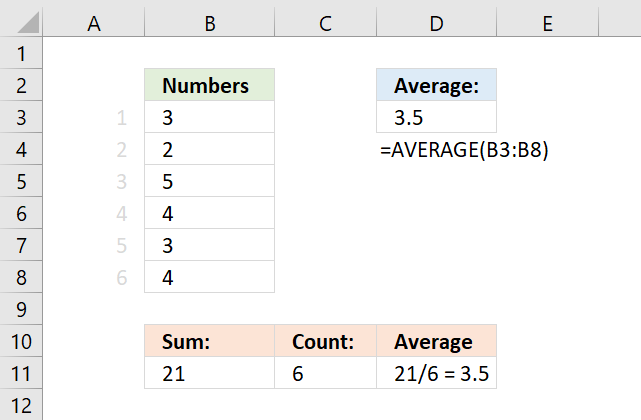Calculates the average of numbers in a cell range. In other words, the sum of a group of numbers and then dividing by the count of those numbers.

## 1. AVERAGE function syntax

The AVERAGE function may have up to 255 arguments, each argument can contain a reference to a single cell, cell range, and/or constants.

You can also use other functions in the arguments, this makes it possible to calculate an average based on a condition or criteria among many other things.

AVERAGE(number1, [number2], ...)

## 2. AVERAGE function arguments

The first argument is required, the remaining arguments are optional.

number1 - Required. numbers or a cell range

[number2] - Optional. Additional arguments up to 255

## 3. How the AVERAGE function works

Here is how the AVERAGE function works: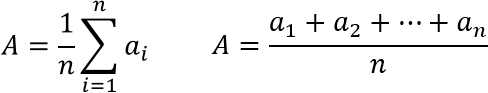## 4. How to average a column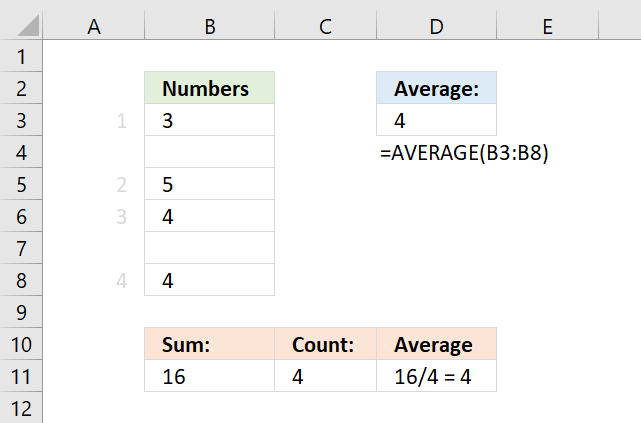This example demonstrates a formula in cell D3 that calculates an average based on a column, the column contains both numerical values and empty cells.

This is not a problem, the AVERAGE function is designed to ignore empty blank cells.

Formula in cell D3:

=AVERAGE(B3:B8)

Cells B4 and B7 are empty, they are not counted. 3 + 5 + 4 + 4 equals 16. 16 / 4 equals 4.

## 5. How to average a column containing some text values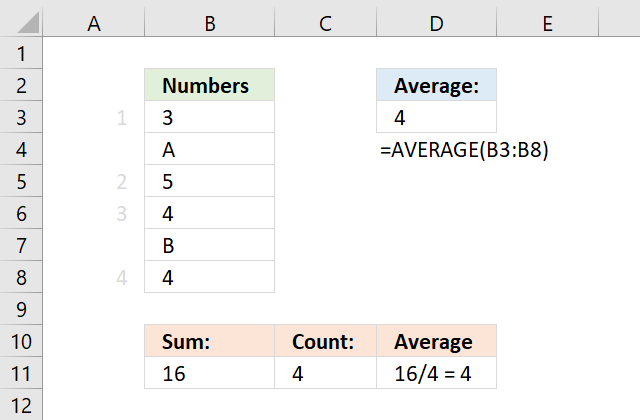This example demonstrates a formula in cell D3 that calculates an average based on a column, the column contains both numerical values and text values.

This is also not a problem, the AVERAGE function is designed to ignore text values, however, keep in mind that error values are not ignored.

Formula in cell D3:

=AVERAGE(B3:B8)

Cells B4 and B7 are empty, they are not counted. 3 + 5 + 4 + 4 equals 16. 16 / 4 equals 4.

## 6. Are zeros included in the AVERAGE function?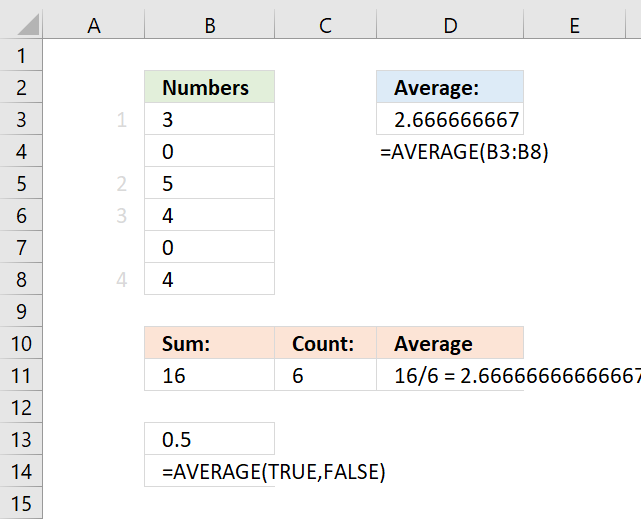This example demonstrates a formula in cell D3 that calculates an average based on values located in a column, the column contains numerical values including some zeros.

The AVERAGE function calculates an average including zeros by default. How to calculate an average and ignore 0 (zeros)

Formula in cell D3:

=AVERAGE(B3:B8)

Cells B4 and B7 are empty, they are not counted. 3 + 5 + 4 + 4 equals 16. 16 / 4 equals 4.

Cells B4 and B7 contain 0 (zeros), they are counted.

## 7. Are boolean values ignored in the AVERAGE function?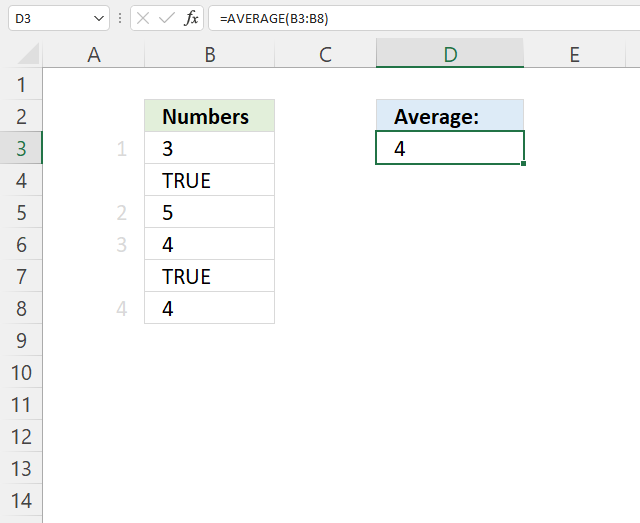Boolean values in a cell range are ignored and not counted shown in cell D3 in the example above.

Formula in cell D3:

=AVERAGE(B3:B8)

3 + 5 + 4 + 4 = 16. 16/4 = 4.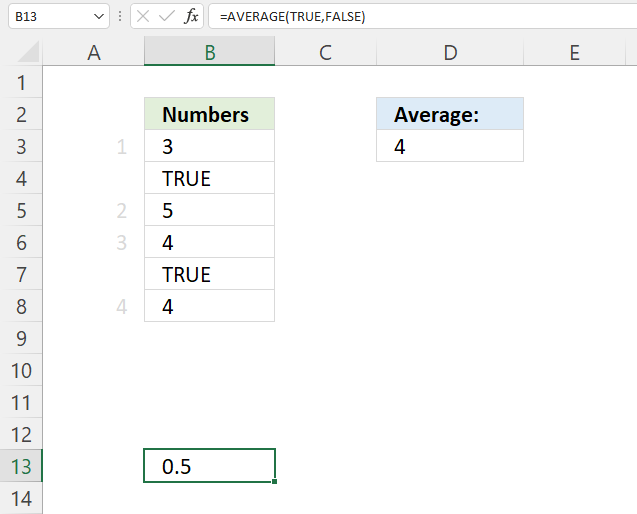Boolean values are ignored by the AVERAGE function, however, they are included if you enter a boolean value as a constant in an argument, see cell B13 above.

The numerical equivalents are:

TRUE = 1
FALSE = 0 (zero)

Formula in cell B13:

=AVERAGE(TRUE, FALSE)

1 + 0 = 1. 1/2 = 0.5 Cell B13 returns 0.5.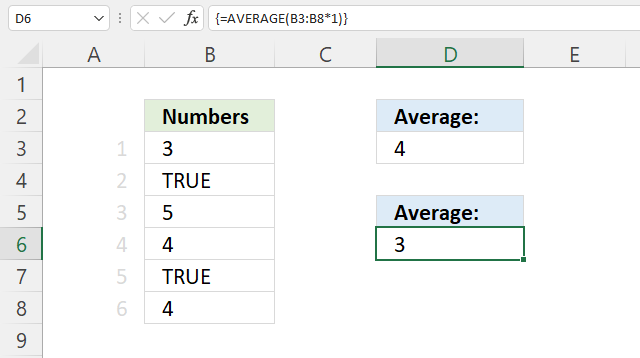To average boolean values multiply the cell range with 1, this converts boolean values to their numerical equivalent.

Array formula in cell D6:

=AVERAGE(B3:B8*1)

### 7.1 How to enter an array formula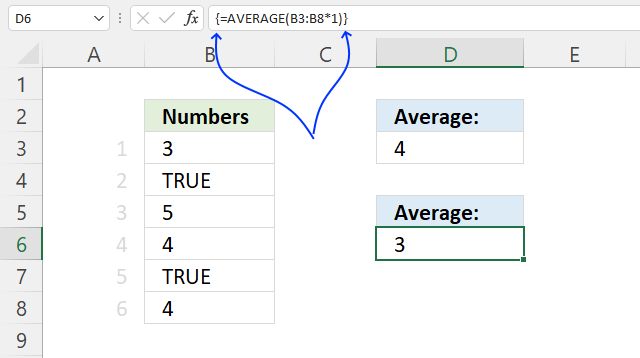To enter an array formula, type the formula in a cell then press and hold CTRL + SHIFT simultaneously, now press Enter once. Release all keys.

The formula bar now shows the formula with a beginning and ending curly bracket telling you that you entered the formula successfully. Don't enter the curly brackets yourself.

### 7.2 Explaining formula in cell D6

#### Step 1 - Convert boolean values

B3:B8*1

becomes

{3; TRUE; 5; 4; TRUE; 4}*1

and returns

{3; 1; 5; 4; 1; 4}.

#### Step 2 - Calculate the average

AVERAGE(B3:B8*1)

becomes

AVERAGE({3; 1; 5; 4; 1; 4})

and returns 3. 3 + 1 + 5 + 4 + 1 + 4 = 18. 18/6 = 3.

## 8. How to average absolute values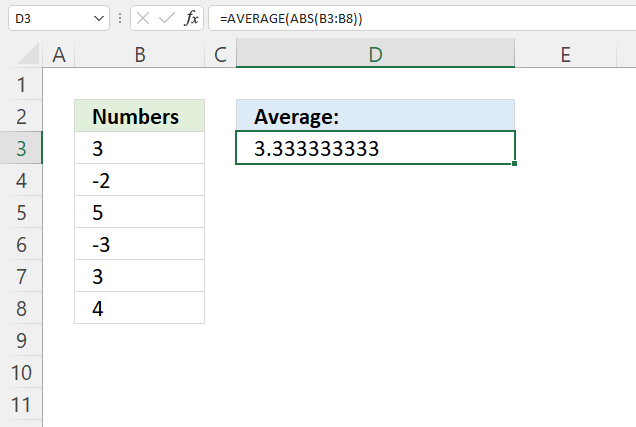The array formula in cell D3 converts numbers in B3:B8 to absolute numbers meaning the minus sign is removed.

Formula in cell D3:

=AVERAGE(ABS(B3:B8))

3 + 2 + 5 + 3 + 3 + 4 equals 20. 20 / 6 = approx. 3.33

How to enter an array formula
<h3id="8">8. Explaining formula

#### Step 1 - Calculate positive numbers

The ABS function converts negative numbers to positive numbers, in other words, the ABS function removes the sign.

ABS(number)

ABS(B3:B8)

becomes

ABS({3; -2; 5; -3; 3; 4})

and returns

{3; 2; 5; 3; 3; 4}.

#### Step 2 - Calculate the average

AVERAGE(ABS(B3:B8))

becomes

AVERAGE({3; 2; 5; 3; 3; 4})

and returns approx. 3.33

## 9. How to calculate an average across sheets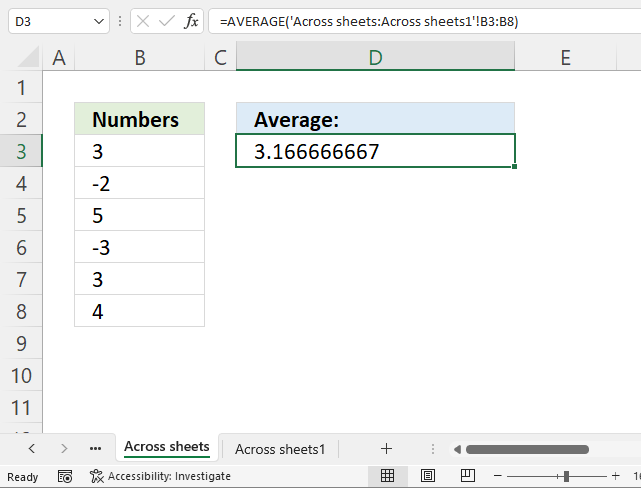In order to calculate an average across worksheets values must be located at the same cell range throughout all worksheets.

Formula in cell D3:

=AVERAGE('Across sheets:Across sheets1'!B3:B8)

The formula uses values from sheets 'Across sheets' and 'Across sheets1' and returns approx. 3.167

Here is how to enter this formula:

1. Double press with left mouse button on cell D3, the prompt appears.
2. Type =AVERAGE(
3. Select cell range B3:B8 with mouse
4. Press and hold SHIFT key.
5. Select tab "Across sheets1".
6. Release SHIFT key.
7. Type )
8. Press Enter.

## 10. How to average by group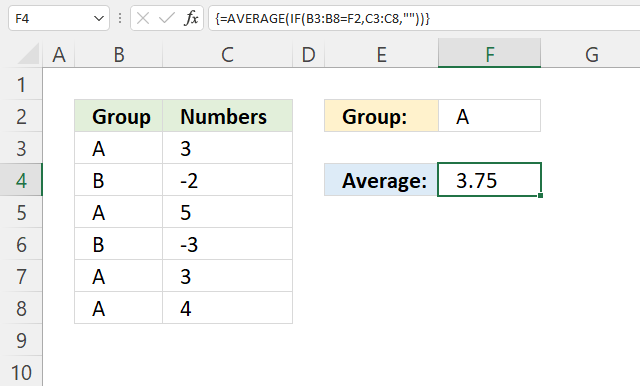The image above demonstrates an array formula that calculates an average based on a condition specified in cell F2.

Array formula in cell D3:

=AVERAGE(IF(B3:B8=F2,C3:C8,""))

How to enter an array formula

### Explaining formula

#### Step 1 - Logical test

The equal sign lets you compare value to value, the result is a boolean value TRUE or FALSE. This will tell us which cells are equal to the condition.

B3:B8=F2

becomes

{"A"; "B"; "A"; "B"; "A"; "A"}="A"

and returns

{TRUE; FALSE; TRUE; FALSE; TRUE; TRUE}.

#### Step 2 - Evaluate IF function

The IF function returns one value if the logical test is TRUE and another value if the logical test is FALSE.

IF(logical_test, [value_if_true], [value_if_false])

IF(B3:B8=F2,C3:C8,"")

becomes

IF({TRUE; FALSE; TRUE; FALSE; TRUE; TRUE},C3:C8,"")

becomes

IF({TRUE; FALSE; TRUE; FALSE; TRUE; TRUE},{3; -2; 5; -3; 3; 4},"")

and returns

{3; ""; 5; ""; 3; 4}.

#### Step 3 - Calculate average

AVERAGE(IF(B3:B8=F2,C3:C8,""))

becomes

AVERAGE({3; ""; 5; ""; 3; 4})

and returns 3.75

Note, the AVERAGEIF and AVERAGEIFS functions are built to handle conditions without the need for an array formula. They are available for Excel 2007 users and later versions, I highly recommend you check them out.

## 11. How to average by month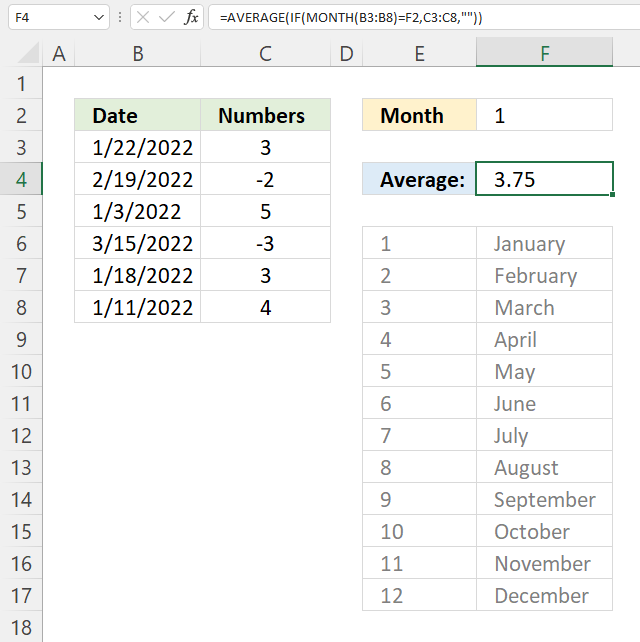Array formula in cell F4:

=AVERAGE(IF(MONTH(B3:B8)=F2,C3:C8,""))

How to enter an array formula

### Explaining formula

#### Step 1 - Calculate month number

The MONTH function returns a number corresponding to the position of a given month in a year. January = 1, ... December = 12.

MONTH(B3:B8)

becomes

MONTH({44583; 44611; 44564; 44635; 44579; 44572})

and returns

{1; 2; 1; 3; 1; 1}.

#### Step 2 - Identify numbers equal to the specified month

The equal sign lets you compare value to value, the result is a boolean value TRUE or FALSE. This will tell us which cells are equal to the condition.

MONTH(B3:B8)=F2

becomes

{1; 2; 1; 3; 1; 1}=F2

becomes

{1; 2; 1; 3; 1; 1}=1

and returns

{TRUE; FALSE; TRUE; FALSE; TRUE; TRUE}.

#### Step 3 - Replace TRUE with corresponding number in C3:C8

The IF function returns one value if the logical test is TRUE and another value if the logical test is FALSE.

IF(logical_test, [value_if_true], [value_if_false])

IF(MONTH(B3:B8)=F2,C3:C8,"")

becomes

IF({TRUE; FALSE; TRUE; FALSE; TRUE; TRUE}, C3:C8, "")

becomes

IF({TRUE; FALSE; TRUE; FALSE; TRUE; TRUE}, {3; -2; 5; -3; 3; 4}, "")

and returns

{3; ""; 5; ""; 3; 4}.

#### Step 4 - Calculate average

AVERAGE(IF(MONTH(B3:B8)=F2,C3:C8,""))

becomes

AVERAGE({3; ""; 5; ""; 3; 4})

and returns 3.75

3 + 5 + 3 + 4 = 15. 15/4 = 3.75

## 12. How to average blank as zero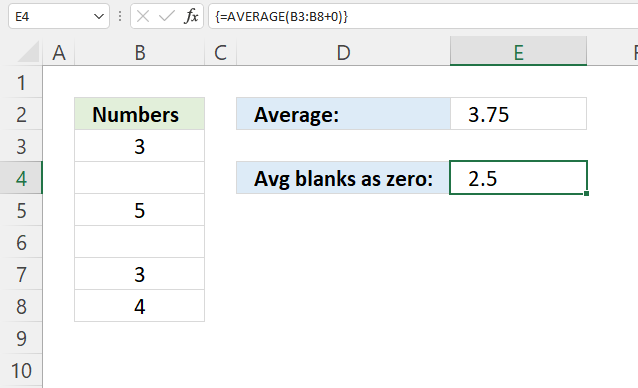Array formula in cell E4:

=AVERAGE(B3:B8+0)

How to enter an array formula

### Explaining formula

#### Step 1 - Add 0 (zero) to values

The plus sign lets you add numbers in Excel, an empty cell converts to a 0 (zero).

B3:B8+0

becomes

{3; ""; 5; ""; 3; 4} + 0

and returns {3; 0; 5; 0; 3; 4}.

#### Step 2 - Calculate average

AVERAGE(B3:B8+0)

becomes

AVERAGE({3; 0; 5; 0; 3; 4})

and returns 2.5

3+0+5+0+3+4 = 15. 15/6 = 2.5

Average without blanks as zeros: 3+5+3+4 = 15. 15/4 = 3.75

## 13. How to average excluding high and low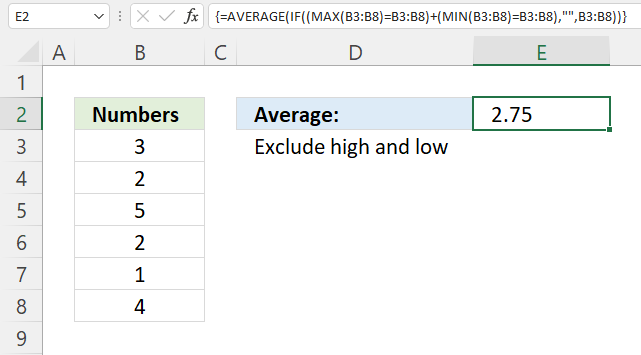Array formula in cell E2:

=AVERAGE(IF((MAX(B3:B8)=B3:B8)+(MIN(B3:B8)=B3:B8),"",B3:B8))

How to enter an array formula

### Explaining formula

#### Step 1 - Calculate the largest value

The MAX function returns the largest value in a cell range or array.

MAX(number1, [number2], ...)

MAX(B3:B8)

becomes

MAX({3; 2; 5; 2; 1; 4})

and returns 5.

#### Step 2 - Compare largest value to B3:B8

The equal sign lets you compare value to value, the result is a boolean value TRUE or FALSE. This will tell us which cells are equal to the largest number.

MAX(B3:B8)=B3:B8

becomes

5={3; 2; 5; 2; 1; 4}

and returns

{FALSE; FALSE; TRUE; FALSE; FALSE; FALSE}.

#### Step 3 - Calculate smallest value

The MIN function returns the smallest number in cell range or array.

MIN(number1, [number2], ...)

MIN(B3:B8)

becomes

MIN({3; 2; 5; 2; 1; 4})

and returns 1.

#### Step 4 - Compare the smallest number to numbers in B3:B8

The equal sign lets you compare value to value, the result is a boolean value TRUE or FALSE. This will tell us which cells are equal to the smallest number.

MIN(B3:B8)=B3:B8

becomes

1= {3; 2; 5; 2; 1; 4}

and returns

{FALSE; FALSE; FALSE; FALSE; TRUE; FALSE}.

#### Step 5 - Add arrays (OR logic)

The plus sign lets you add values and also arrays, this creates OR logic between the two arrays containing boolean values.

TRUE + TRUE = TRUE
TRUE + FALSE = TRUE
FALSE + TRUE = TRUE
FALSE + FALSE = FALSE

One more thing, the boolean values are converted into their numerical equivalents when you add the arrays.

TRUE = 1 or in fact any number except zero
FALSE = 0 (zero)

(MAX(B3:B8)=B3:B8)+(MIN(B3:B8)=B3:B8)

becomes

{FALSE; FALSE; TRUE; FALSE; FALSE; FALSE} + {FALSE; FALSE; FALSE; FALSE; TRUE; FALSE}

and returns {0; 0; 1; 0; 1; 0}.

#### Step 6 - Replace 0 (zero) with corresponding value

The IF function returns one value if the logical test is TRUE and another value if the logical test is FALSE.

IF(logical_test, [value_if_true], [value_if_false])

IF((MAX(B3:B8)=B3:B8)+(MIN(B3:B8)=B3:B8),"",B3:B8)

becomes

IF({0; 0; 1; 0; 1; 0},"",B3:B8)

becomes

IF({0; 0; 1; 0; 1; 0},"", {3; 2; 5; 2; 1; 4})

and returns {3; 2; ""; 2; ""; 4}.

#### Step 7 - Calculate average

AVERAGE(IF((MAX(B3:B8)=B3:B8)+(MIN(B3:B8)=B3:B8),"",B3:B8))

becomes

AVERAGE({3; 2; ""; 2; ""; 4})

and returns 2.75

3 +2 + 2 + 4 = 11. 11/4 = 2.75

## 14. How to exclude some cells from average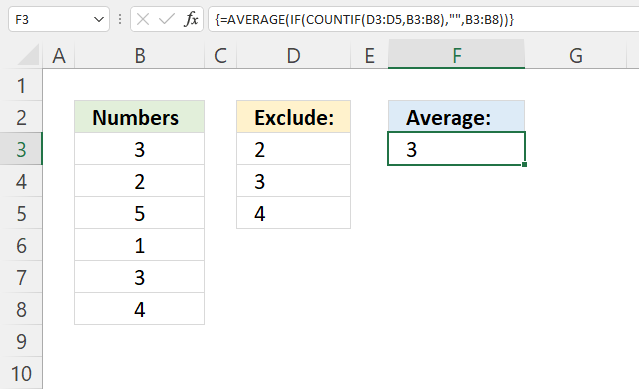Array formula in cell F3:

=AVERAGE(IF(COUNTIF(D3:D5, B3:B8), "", B3:B8))

How to enter an array formula

### Explaining formula

#### Step 1 - Identify numbers based on criteria

The COUNTIF function calculates the number of cells that is equal to a condition. This will tell us where the excluded cells are.

COUNTIF(rangecriteria)

COUNTIF(D3:D5, B3:B8)

becomes

COUNTIF({2;3;4},{3;2;5;1;3;4})

and returns

{1; 1; 0; 0; 1; 1}.

#### Step 2 - Replace 1 with nothing and 0 (zero) with number

The IF function returns one value if the logical test is TRUE and another value if the logical test is FALSE.

IF(logical_test, [value_if_true], [value_if_false])

IF(COUNTIF(D3:D5, B3:B8), "", B3:B8)

becomes

IF({1; 1; 0; 0; 1; 1}, "", B3:B8)

becomes

IF({1; 1; 0; 0; 1; 1}, "", {3; 2; 5; 1; 3; 4})

and returns

{""; ""; 5; 1; ""; ""}.

#### Step 3 - Calculate average

AVERAGE(IF(COUNTIF(D3:D5, B3:B8), "", B3:B8))

becomes

AVERAGE({""; ""; 5; 1; ""; ""})

and returns 3. 5+1 = 6. 6/2 = 3

## Get Excel *.xlsx file

### Get the Excel fileAVERAGE-function.xlsx